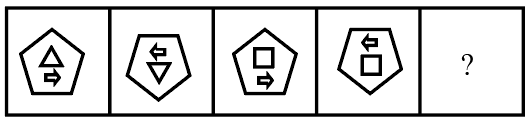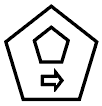Question 10

# Select the figure that can replace the question mark (?) in the following series.Solution

The figure rotate 180$$\degree$$ in each step so, next figure,$$\therefore$$ The correct answer is option D.

• Free SSC Study Material - 18000 Questions
• 230+ SSC previous papers with solutions PDF